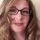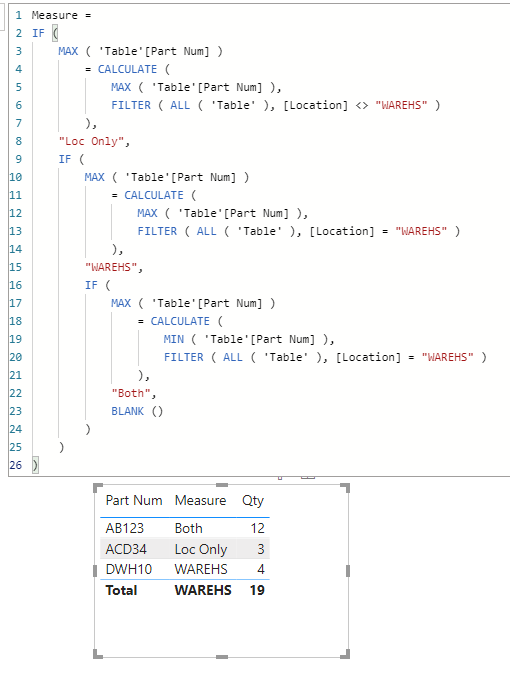cancel
Showing results for
Did you mean:## Determine if a value exists in one column, when grouping by another column

I'm probably asking or searching wrong, but have been struggling to solve this issue for about a week at this point.
I am using a DirectQuery on a published report, and therefore I cannot unpivot the data in Power Query. I need to find a measure solution (I believe)

My data table looks like this:
Part Num   |   Location   |    Qty
AB123        |  ALOC1       |    10
AB123        | WAREHS     |     2
ACD34       |   BLOC1       |     3
DWH10      | WAREHS     |     4

I need a solution that looks at all the Part Num values and if "WAREHS" is one of the Locations, then ="Both".
If "WAREHS" is Only Location = "Only WARE" and if WAREHS is not a Location then  = "Loc Only".

End result desired:
Part Num   |   Sort Loc   |    Qty
AB123        |  Both          |    12
ACD34       |  Loc Only    |     2
DWH10     |  Only WARE |    4

The main trouble I'm having is if I apply a filter to another category and that filters the Part Num field in any way, this evaluation stops working as now the Locations do not apply.  I need the evaluation to always check for WAREHS and not filter the Part Num field at all.

Thanks

Jessica

1 ACCEPTED SOLUTIONSuper User

@JessieF Try:

``````Sort Loc Measure =
VAR __CountWareHS = COUNTROWS(FILTER('Table',[Location]="WAREHS"))
VAR __CountLoc = COUNTROWS(SUMMARIZE('Table',[Location]))
RETURN
SWITCH(TRUE(),
__CountWareHS >= 1 && __CountLoc = 1,"Only WARE",
__CountWareHS >= 1 && __CountLoc > 1,"Both",
"Loc Only"
)``````

Become an expert!: Enterprise DNA
External Tools: MSHGQM
Latest book!:
Mastering Power BI 2nd EditionDAX is easy, CALCULATE makes DAX hard...
4 REPLIES 4Community Support

Hi, @JessieF

You can try the following methods.

1. Calculated column

``````Sort Loc =
IF (
[Part Num]
= CALCULATE (
MAX ( 'Table'[Part Num] ),
FILTER ( 'Table', [Location] <> "WAREHS" )
),
"Loc Only",
IF (
[Part Num]
= CALCULATE (
MAX ( 'Table'[Part Num] ),
FILTER ( 'Table', [Location] = "WAREHS" )
),
"WAREHS",
IF (
[Part Num]
= CALCULATE (
MIN ( 'Table'[Part Num] ),
FILTER ( 'Table', [Location] = "WAREHS" )
),
"Both",
BLANK ()
)
)
)
``````1. Measure

``````Measure =
IF (
MAX ( 'Table'[Part Num] )
= CALCULATE (
MAX ( 'Table'[Part Num] ),
FILTER ( ALL ( 'Table' ), [Location] <> "WAREHS" )
),
"Loc Only",
IF (
MAX ( 'Table'[Part Num] )
= CALCULATE (
MAX ( 'Table'[Part Num] ),
FILTER ( ALL ( 'Table' ), [Location] = "WAREHS" )
),
"WAREHS",
IF (
MAX ( 'Table'[Part Num] )
= CALCULATE (
MIN ( 'Table'[Part Num] ),
FILTER ( ALL ( 'Table' ), [Location] = "WAREHS" )
),
"Both",
BLANK ()
)
)
)
``````Best Regards,

Community Support Team _Charlotte

If this post helps, then please consider Accept it as the solution to help the other members find it more quickly.This was similar to solutions I was trying and unable to get it to work properly. I think there is a data type issue, but since I cannot change transform the data, I am having difficulty.  I appreciate your time to answer my inquiry.Super User

@JessieF Try:

``````Sort Loc Measure =
VAR __CountWareHS = COUNTROWS(FILTER('Table',[Location]="WAREHS"))
VAR __CountLoc = COUNTROWS(SUMMARIZE('Table',[Location]))
RETURN
SWITCH(TRUE(),
__CountWareHS >= 1 && __CountLoc = 1,"Only WARE",
__CountWareHS >= 1 && __CountLoc > 1,"Both",
"Loc Only"
)``````

Become an expert!: Enterprise DNA
External Tools: MSHGQM
Latest book!:
Mastering Power BI 2nd EditionDAX is easy, CALCULATE makes DAX hard...@Greg_Deckler   Thank you so much for the assistance. This worked with my dataset.  I appreciate the help very much.

Jessie.